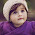### Capacitance of Three Core Cables

In three core cables, capacitance play an important role because in such cables capacitances exist between the cores as well as each core and the sheath. These capacitances are dominating as the dielectric constant of the dielectric used in cables is much more than the air. The capacitances are shown in the Fig. 1.Fig. 1
The core to core capacitances are denoted as Cc while core to sheath capacitance are denoted as Cs.
The core to core capacitances Cc are in delta and can be represented in the equivalent star as shown in the Fig. 2.Fig. 2
The impedance between core 1 and the star point, Z can be obtained as,
If star point is assumed to be at earth potential and if sheath is also earthed then the capacitance of each conductor to neutral is,
1.1 Measurement of Cand C
The total capacitance is not easy to calculate but by actual practical measurement C and C can be determined.
Practical measurement involves two cases :
Case 1 : The core 2 and 3 are connected to sheath.
Thus the C between cores 2 and 3 and C between cores 2, 3 and sheath get eliminated as shown in the Fig. 3.
All the three capacitances are now in parallel across core 1 and the sheath.
The capacitance of core 1 with sheath is measured practically and denoted by Ca.
C = C + 2C                                 ............(1)
Case 2 : All the three cores are bundled together.
This eliminates all the core-core capacitances. This is shown in the Fig. 5.Fig. 5
The capacitances C are in parallel between the common core and sheath.
This capacitance is practically measured and denoted as Cb.
Cb   = 3 C                               ...........(2)
Solving (1) and (2) simultaneously,
C = (Cb /3) + 2C
C = (Ca /2)-(Cb /2)   and C = Cb /3
Thus both the capacitances can be determined.
C = C + 3C =(Cb/3) + 3((Ca /2) -(Cb /2))

1.2 Capacitance of Three Core Cable
There is one empirical formula to calculate the capacitance of a three core belted cable, stated by Simon. It is applicable for the circular conductors. The formula gives the capacitance of a three core cable to neutral per phase per kilometer length of the cable. The formula is given as,
Where        εr = Relative permittivity of the dielectric
d = Conductor diameter
t = Belt Insulation thickness
T = Conductor insulation thickness
The formula can be used when the test results are not available. This gives approximate value of the capacitance. If εr is not given, it can be assumed to be 3.5. It must be remembered that all the values of d, t and T must be used in the same units while using the formula.
Example : A three core cable has core diameter 0f 2 cm and core to core distance of 4 cm. The dielectric material has relative permittivity of 5. Compute the capacitance of this cable per phase per km. Thickness of the conductor insulation is 1 cm and that of belt insulation is 0.5 cm.
Solution : d = 2 cm ,  εr = 5,   T = 1 cm,   t = 0.5 cm
Use the empirical formula as the test results are not given.

Read examples on three core cables

1.Wonderful ideas! You are providing the different kinds of content is very useful for me and I gain more details from your article. I am always following your blog and keep posting...
Spark Training in Chennai
Spark Training
Embedded System Course Chennai
Linux Training in Chennai
Social Media Marketing Courses in Chennai
Power BI Training in Chennai
Tableau Training in Chennai
Oracle DBA Training in Chennai

2.Great info. The content you wrote is very interesting to read. This will loved by all age groups.
ReactJS Training in Chennai
ReactJS Training
ReactJS course
ccna Training in Chennai
Salesforce Training in Chennai
Angularjs Training in Chennai

3.Nice post. I learned some new information. Thanks for sharing.

Article submission sites
Technology

4.